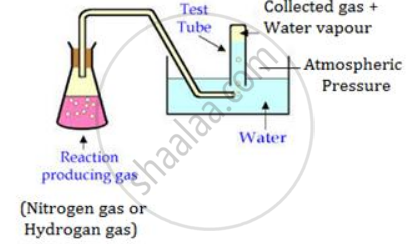# What is Meant by Aqueous Tension? How is the Pressure Exerted by a Gas Corrected to Account for Aqueous Tension? - Chemistry

What is meant by aqueous tension? How is the pressure exerted by a gas corrected to account for aqueous tension?

#### Solution

Gases such as nitrogen and hydrogen are collected over water as shown in the diagram. When the gas is collected over water, the gas is moist and contains water vapour. The total pressure exerted by this moist gas is equal to the sum of the partial pressures of the dry gas and the pressure exerted by water vapour. The partial pressure of water vapour is also known as aqueous tension.Ptotal = Pgas + Pwater vapour

Pgas = Ptotal- Pwater vapour

Actual pressure of gas = Total pressure - Aqueous tension

Concept: The Gas Laws
Is there an error in this question or solution?

#### APPEARS IN

Selina Concise Science Chemistry 1 Class 9 ICSE
Chapter 7 Study of Gas Laws
Exercise 7 (A) | Q 20 | Page 126